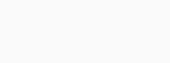# Learn About Disjoint and Equal Sets With Properties and Examples

Share With Friends or Family

## Learn About Disjoint and Equal Sets With Properties and ExamplesA set is a well-defined collection of objects. These objects are referred to as set elements. Sets are classed according to the number of elements they include. A Set is a grouping of distinct elements of the same type. For example, a fruit basket, a tea set, a set of real numbers, natural numbers, and so forth.

Let’s discuss two such important types of sets i.e., Disjoint and Equal Sets which are regularly asked in various competitive exams.

## Disjoint Sets

Disjoint Sets are two sets that do not share any elements. Set A={2,3}  and set B={4,5} for example, are disjoint sets. However, set C={3,4,5} and {3,6,7} are not disjoint because both sets C and D include 3 as a common element.

Disjoint sets are formed when the intersection of two sets is a null or empty set. As a result, if A and B are two disjoint sets, then;

A ∩ B = ϕ

The definition of disjoint sets can be expanded to a family of sets (Ai)i I I: the family is disjoint if Ai ⋂ Aj = Φ whenever i ≠ j. When it comes to family notation, it can be described as the notion of pairwise disjoint or mutually disjoint is often expressed in a significantly different way, in that repeated identical members are permitted.

### Pairwise Disjoint Sets

If any two sets in a group are disjoint, the group is said to be pairwise disjoint. It’s also known as mutually disjoint sets. Let A be any group of sets, and P and Q be two sets within A, i.e. P, Q ∈ A. Then A is said to be a pairwise disjoint if and only if P ≠ Q. As a result, P ∩ Q = ϕ.

### Important Notes on Disjoint Sets

Let’s discuss a few points to keep in mind when studying disjoint sets.

• Disjoint sets have no common elements since the intersection set operation between them always returns a null or empty set.
• When two sets intersect and produce a null set, they are said to be pairwise disjoint.

## Equal Sets

Equal sets are those that have the same cardinality and have all equal members. They are generally represented by a capital letter and the braces ‘{}’. In other words, two or more sets are considered equal if they include the same elements and have the same number of elements. Set A = {1, 2, 3, 4, 5} and B = {1, 2, 3, 4, 5} as an example. Then sets A and B are considered equal sets because their elements and cardinality are the same.

Now, two sets are said to be unequal if their elements are not all the same, whereas sets with the same number of items are called equivalent. For instance, suppose A = {1, 2, 3, 4, 5}, C = {2, 4, 6, 7, 9} and D = {2, 5, 6}. Sets A and C have the same number of elements, but not all of them are equal. As a result, A and C are equivalent sets. Sets A and D no longer have the same cardinality, and their elements are not equal. As a result, sets A and D are unequal. The number of elements and similarity of elements in the two sets can be used to determine whether the two sets are equal or equivalent.

### Properties of Equal Sets

We now understand the concept of equal sets. Following that, we will look at some of its key properties that will aid in understanding and identification:

• The equality of the two sets is unaffected by the order of the components.
• Equal sets have the same cardinality or the same number of elements.
• When two sets are subsets of one another, the set notation is A B and B ⊆ A, and the two sets are equal. A = B.
• All elements in equal sets must be equal.
• The power set of equal sets has the same cardinal number.
• Equal and equivalent sets share the attribute of having an equal number of elements.
• The reverse is not true: all equal sets are equivalent sets.